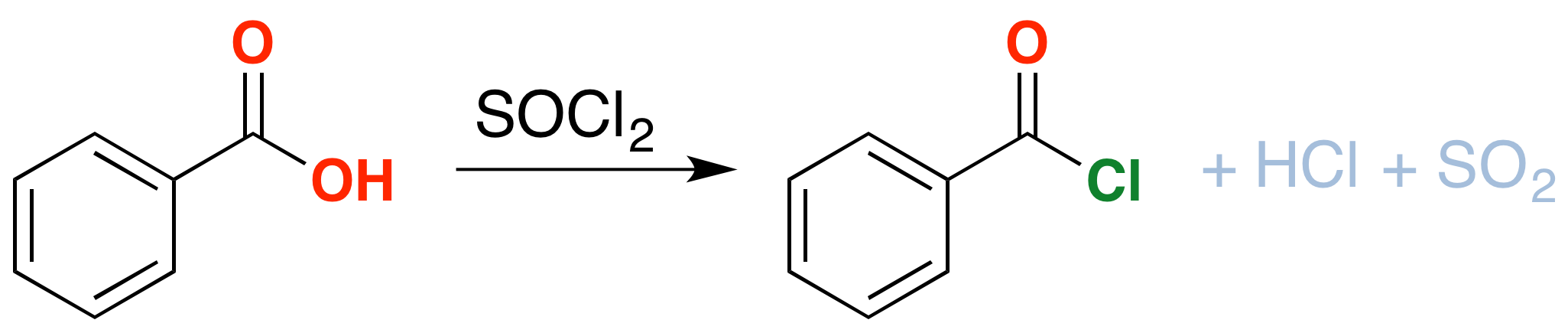Thionyl Chloride

If There is one thing you learn how to do well in Org 1, it’s make alcohols. Let’s count the ways: hydroboration, acid-catalyzed hydration, oxymercuration for starters, and then substitution of alkyl halides with water or $$HO^-$$. If you want to extend it even further, There is dihydroxylation (to make diols) using $$OsO_4$$ or cold $$KMnO_4$$, and even opening of epoxides under acidic or basic conditions to give alcohols.

There is just one issue here and it comes up once you try to use alcohols in synthesis. Let’s say you want to use that alcohol in a subsequent substitution step, getting rid of the $$HO^-)\ and replacing it with something else. See any problems with that? Remember that good leaving groups are weak bases – and the hydroxide ion, being a strong base, tends to be a pretty bad leaving group. So what can we do? What you want to do is convert the alcohol into a better leaving group. One way is to convert the alcohol into a sulfonate ester – we talked about that with \( TsCl$$ and $$MsCl$$. Alternatively, alcohols can be converted into alkyl chlorides with thionyl chloride ($$SOCl_2$$). This is a useful reaction, because the resulting alkyl halides are versatile compounds that can be converted into many compounds that are not directly accessible from the alcohol itself.If you take an alcohol and add thionyl chloride, it will be converted into an alkyl chloride. The byproducts here are hydrochloric acid ($$HCl$$) and sulfur dioxide ($$SO_2$$). Note: there are significant differences in how this reaction is taught at different schools. Consult your instructor to be 100% sure that this applies to your course). See post here

Example $$\PageIndex{1}$$: Conversion of Alcohols to Alkyl ChloridesThere is one important thing to note here: see the stereochemistry? It’s been inverted.*(white lie alert – see below) That’s an important difference between $$SOCl_2$$ and TsCl, which leaves the stereochemistry alone. We’ll get to the root cause of that in a moment, but in the meantime, can you think of a mechanism which results in inversion of configuration at carbon?

As an extra bonus, thionyl chloride will also convert carboxylic acids into acid chlorides (“acyl chlorides”). Like alcohols, carboxylic acids have their limitations as reactants: the hydroxyl group interferes with many of the reactions we learn for nucleophilic acyl substitution (among others). Conversion of the OH into Cl solves this problem.

Example $$\PageIndex{2}$$: Conversation of Carboxylic Acids to Acid ChloridesMechanism

As you might have guessed, conversion of alcohols to alkyl halides proceeds through a substitution reaction – specifically, an $$S_N2$$ mechanism. The first step is attack of the oxygen upon the sulfur of $$SOCl_2$$, which results in displacement of chloride ion. This has the side benefit of converting the alcohol into a good leaving group: in the next step, chloride ion attacks the carbon in $$S_N2$$ fashion, resulting in cleavage of the C–O bond with inversion of configuration. The $$HOSCl$$ breaks down into $$HCl$$ and sulfur dioxide gas, which bubbles away.

Formation of Alkyl Chlorides

Since the reaction proceeds through a backside attack ($$S_N2$$), there is inversion of configuration at the carbonThe mechanism for formation of acid chlorides from carboxylic acids is similar. The conversion of caboxylic acids to acid chlorides is similar, but proceeds through a [1,2]-addition of chloride ion to the carbonyl carbon followed by [1,2]-elimination to give the acid chloride, $$SO_2$$ and $$HCl$$In the laboratory

Like many sulfur-containing compounds, thionyl chloride is noseworthy for its pungent smell. Thionyl chloride has a nauseating sickly-sweet odor to it that imprints itself forever upon your memory. One accident that occurred during my time as a TA involved a student dropping a flask with 5 mL of thionyl chloride into a rotovap bath outside the fume hood. The cloud of $$SO_2$$ and $$HCl$$ that formed cleared the teaching lab for half an hour, so you can imagine what thionyl chloride would do if exposed to the moisture in your lungs. Treat with caution, just as you would if you were working with phosgene.

*Here’s the white lie. Although it’s often taught that $$SOCl_2$$ leads to 100% inversion of configuration, in reality it’s not always that simple. Inversion of configuration with $$SOCl_2$$ is very solvent dependent. Depending on the choice of solvent, one can get either straight inversion, or a mixture of retention and inversion. For the purposes of beginning organic classes, most students can ignore this message.